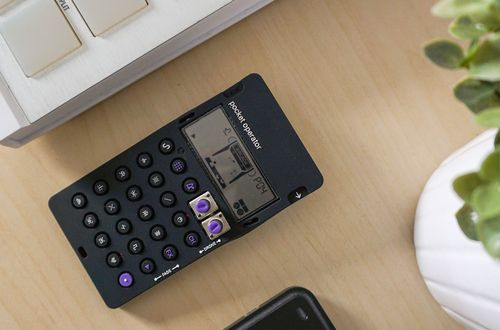# How to do reverse percentages

Want to find out how to do reverse percentages? We've provided a step-by-step guide of how to do reverse percentages to teach you everything you need to know.## Step 1: Add the percentage to 100

This means that if we want to look for the original number given that if we increase it by 15%, the result is 92.

## Step 2: Turn the percentage into a decimal

Change the percentage from Step 1 to decimal by moving two decimal places to the left.

## Step 3: Divide by the decimal form

Divide the given final value by the decimal form from Step 2. So, to find the original number, we can divide 92 by 1.15.

## Examples of how to reverse percentages

Q1) Nathaniel bought a pair of gaming console at 75% its original price. If he bought the console for \$240, how much is the gaming console’s original price?

To find the original cost of the console, we first change 75% to decimal.

75%=0.75

We can then divide \$240 by 0.75 to find the original price of the console.

240÷0.75=320

This means that the gaming console originally costs \$320.

Q2) Jonathan decided to reduce the prices at his coffee shop by 15% to support students as they prepare for their finals. If one medium cup of American now costs \$1.70, how much does one cup cost before Jonathan’s promotion?

We first subtract 100 from 15 100-15=85%

To find the original cost, we have to change 85% to decimal.

85%=0.85

Divide the new cost by 0.85, we have

1.70÷0.85=2

That’s why one cup of Americano used to cost \$2.00.

Q3) Charlie was not satisfied by his average score last semester but he plans to aim for a score 20% higher than that. If his new average score set for semester is 96, what was his previous score last term?

Since we want to find the original value after increasing it by 20%, we add 100 and 20 100+20=120%

We then change 120% to decimal by moving two decimal places to its left.

120%=1.20

Divide 96 by 1.20 to find Charlie’s previous score.

96÷1.20=80

This means that Charlie’s average last semester was 80.

#### Join today

The fastest way to practice

Unlock our complete testing platform and improve faster that ever.